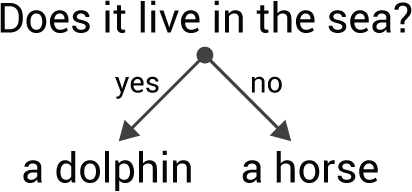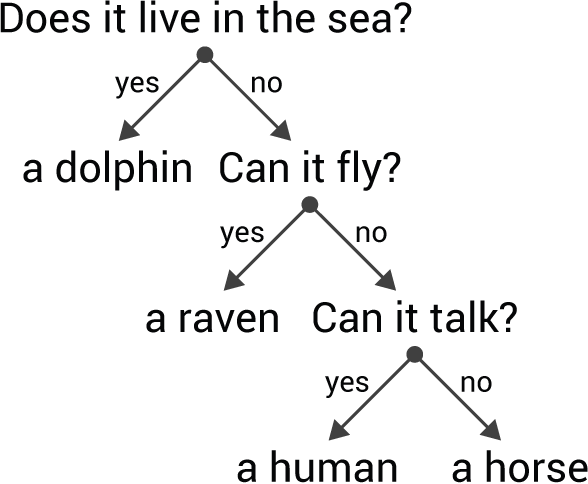## Animals

The following Animals game tries to guess the animal you are thinking of. If it doesn't know the animal it learns it by prompting you to add it to the database.

9th May 2020: Updated the program to take advantage of the format function in uLisp 3.2.

### Introduction

This program has been used in many programming tutorials because it's fun and simple to implement, and demonstrates how a computer can interactively build a database with input from the user. It can be written quite elegantly in uLisp by taking advantage of the language's string and list handling.

It demonstrates some of the new features in uLisp 1.6, but needs more memory than is available on an Arduino Uno or ATmega328. I recommend an Arduino Mega 2560 or ATmega1284-based board.

The same principle could be used to build simple expert systems to debug circuits, or identify electronic components.

Note: To run this program using the Serial Monitor from the Arduino IDE you need to make sure that the line ending option is set to Newline.

### Description

The Animals game tries to guess the animal you are thinking of. To play the game you simply type:

`> (go)`

The program then asks a series of questions, such as:

`Does it live in the sea?`

to which you answer y or n as appropriate. Eventually it makes a guess at the animal:

`Is it a horse?`

If it was correct the game ends. However, if you answered n the program prompts you to enter your animal (start it with a lower-case letter):

`What were you thinking of? a raven`

You are then prompted to add a question to the tree (start with a capital letter and end with a question mark):

`Give me a yes/no question to distinguish between a raven and a horse: Can it fly?`

Finally the program needs to know the correct answer for the new animal:

`What would the answer be for a raven? Y`

As a result of this interaction the new animal has been added to the database, and the next time you play the program will know about the new animal.

### How the program works

The database is represented as a tree of questions and animals. The program starts with one question and two animals:The tree is represented as a list of three items:

`(question yes-answer no-answer)`

So for the tree shown above the list will be:

`("Does it live in the sea?" "a dolphin" "a horse")`

The database tree is stored in a variable called data, so we'll start by defining:

`(defvar *data* '("Does it live in the sea?" "a dolphin" "a horse"))`

After it has been trained with two more animals the tree might look like this:Here's the Lisp representation, indented to make the structure clearer:

```("Does it live in the sea?"
"a dolphin"
("Can it fly?"
"a raven"
("Can it talk?"
"a human"
"a raven")))```

The animals program is built out of these four separate functions:

• run asks a question, or makes a guess, depending on where it is in the tree.
• try makes a guess.

Although we could have combined some of these functions, the advantage of breaking it down into small, separate building-blocks is that we can test each function independently.

### Utilities

First we define a useful utility function yes-no, which reads a symbol and returns t if it's Y:

```(defun yes-no ()

```(defun add (animal new)
(format t "~%Give me a yes/no question to distinguish between ~a and ~a: " new animal)
(format t "~%What would the answer be for ~a ? " new)
(if (yes-no)
(list question new animal)
(list question animal new))))```

This takes two parameters: an existing animal, and a new animal. It prompts for a question, and the correct answer for the new animal, and returns a list of the question and answers in the correct order. For example:

`(add "a cat" "a dog")`

will prompt:

`Give me a yes/no question to distinguish between a dog and a cat: `

and

`What would the answer be for a dog?`

and returns the appropriate list; for example

`("Does it bark?" "a dog" "a cat")`

### Making a guess

The next procedure, try, is called when we've reached an animal on the tree. It takes the animal as its parameter, and guesses that as the answer. If it's correct it simply returns the animal. If it's incorrect, it calls add to create a list containing a new question and the two answers.

```(defun try (animal)
(format t "~%Is it ~a ?" animal)
(cond
((yes-no) (printlist "Ho ho!") animal)
(t (format t "~%What were you thinking of? ")
```

The next procedure, ask, is called when we're at a question on the tree. It prompts with the question, and depending on whether the answer is Y or N it calls run on the left branch or right branch of the tree respectively.

```(defun ask (tree)
(format t "~%~a " (first tree))
(if (yes-no)
(list (first tree) (run (second tree)) (third tree))
(list (first tree) (second tree) (run (third tree)))))```

It returns the complete tree, modified to add a new animal if one was added.

### The main procedure - run

Finally here's the main procedure, run. It is called with the current position on the tree as the parameter, and simply calls ask if the parameter is a list, indicating that more questions need to be asked, or try if it's an animal, indicating that we're at the bottom of a branch.

```(defun run (tree)
(if (listp tree)
(try tree)))```

To save the modified tree we need to save it back as *data*. Here's a routine go that does that for us:

```(defun go ()
(setq *data* (run *data*))
nil)```

### Saving the database

After playing the game a few times you can save the database with:

`(save-image)`

`(load-image)`